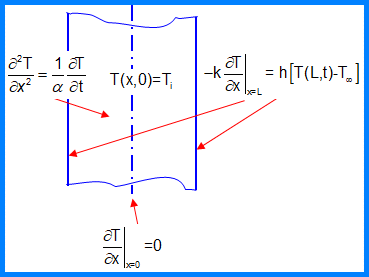# Analytical Solution for Transient Conduction in a Plane Wall

UPDATED: 01/27/2023

This workbook implements the separation-of-variables solution for transient conduction in a slab (plane wall) subject to a sudden change in thermal conditions at its surface.    It uses the analytical solution for a plane wall and calculates numerical values as a function of Biot (= hL/k) and Fourier (= α T/L²) numbers.Schematic for transient conduction in a plane wall. The outside surface on both sides is subject to convection given by Newton’s Law of Cooling (q” = hΔT).  A symmetry condition is applied at the centerline.

Note: because of the difficulties associated with this method (including the solution of an equation involving transcendental functions), the usual procedure is to truncate the series with just one term and graph the resulting solutions (as in the Heisler Charts).   The analytical solutions for the infinite cylinder and the sphere are even messier.   This solution was created to check plain wall results from our HTTonedt Visual Studio executable.  Additional pages solve the characteristic equation for a similar scenario in the infinite cylinder and sphere, also using Excel’s Goal Seek capability.